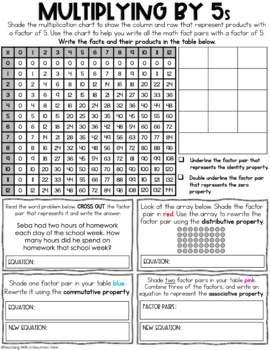digital

# Multiplication Fact Practice | Google Classroom | Distance LearningSubject
Resource Type
Format
Zip (18 MB|25 pages)Google Apps™
Standards
\$4.95
\$4.95The Teacher-Author indicated this resource includes assets from Google Workspace (eg. docs, slides, etc.).

### Description

Are your students ready to master multiplication? I designed this multiplication fact practice resource for students to master their strategies, facts, and multiplication properties.

This resource includes:

• Multiplication Properties Quick Reference Guide
• Ways to Show Multiplication Quick Reference Guide
• Printable Fact and Strategies Practice Book for facts 2-12
• DIGITAL, interactive version in Google Slides
• Cover Pages to make student booklets
Differentiation: This resource includes two options for use: One set reviews properties and strategies. A second version does not include the properties for easy scaffolding and differentiation. Please see the preview for more information.

The Version WITH Multiplication Properties Reviews:

• Identity Property of Multiplication
• Zero Property of Multiplication
• Commutative Property of Multiplication
• Associative Property of Multiplication
• Distributive Property of Multiplication (using arrays)

BOTH Versions Review:

• Using a multiplication chart to find patterns and products
• Creating arrays
• Using a Number Line
• Equal Groups
• Skip Counting
• Multiplication Word Problems

This resource is perfect for teaching, reteaching, practicing, or mastering multiplication math facts. You can use it as a teaching tool and complete it as you learn about the properties and ways to show multiplication, or students can complete it as a review to practice math facts.

Answer Key Note: Please note that for many of the tasks, students select the fact they want to model. For this reason, a complete answer key was not possible. I did, however, include an answer key for all the math facts and the tasks that had concrete answers.

Looking for even more Multiplication Resources?

Total Pages
25 pages
Included
Teaching Duration
N/A
Report this Resource to TpT
Reported resources will be reviewed by our team. Report this resource to let us know if this resource violates TpT’s content guidelines.

### Standards

to see state-specific standards (only available in the US).
Multiply or divide to solve word problems involving multiplicative comparison, e.g., by using drawings and equations with a symbol for the unknown number to represent the problem, distinguishing multiplicative comparison from additive comparison.
Interpret a multiplication equation as a comparison, e.g., interpret 35 = 5 × 7 as a statement that 35 is 5 times as many as 7 and 7 times as many as 5. Represent verbal statements of multiplicative comparisons as multiplication equations.
Identify arithmetic patterns (including patterns in the addition table or multiplication table), and explain them using properties of operations. For example, observe that 4 times a number is always even, and explain why 4 times a number can be decomposed into two equal addends.
Fluently multiply and divide within 100, using strategies such as the relationship between multiplication and division (e.g., knowing that 8 × 5 = 40, one knows 40 ÷ 5 = 8) or properties of operations. By the end of Grade 3, know from memory all products of two one-digit numbers.
Apply properties of operations as strategies to multiply and divide. Examples: If 6 × 4 = 24 is known, then 4 × 6 = 24 is also known. (Commutative property of multiplication.) 3 × 5 × 2 can be found by 3 × 5 = 15, then 15 × 2 = 30, or by 5 × 2 = 10, then 3 × 10 = 30. (Associative property of multiplication.) Knowing that 8 × 5 = 40 and 8 × 2 = 16, one can find 8 × 7 as 8 × (5 + 2) = (8 × 5) + (8 × 2) = 40 + 16 = 56. (Distributive property.)